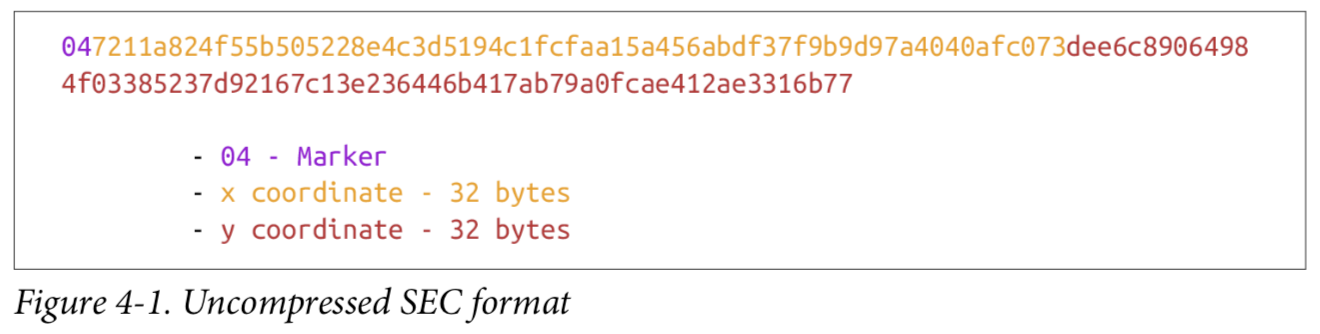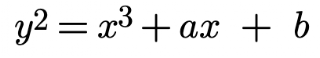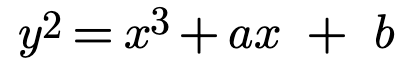## Posts

Showing posts from May, 2022

### Bitcoin Programming: PrivateKey, PublicKey & SignatureIn this writing, is about to serialization, how to transmit PrivateKey, PubKey & Signature to other computers on the network, or even to disk. There will be 2 types SEC,  Standards for Efficient Cryptography. Compressed and Uncompressed. With form provided SEC form for given point P = (x,y) is generate as following: 1.  Start with the prefix byte, which is 0x04. 2.  Next, append the x coordinate in 32 bytes as a big-endian integer. 3.  Next, append the y coordinate in 32 bytes as a big-endian integer. Prefix or marker. '04' meaning Uncompressed, '03' Compressed Odd ending and '02' Compressed Even ending. These are the format for PubKey. Signature itself, there is DER Signatures and format is like following: 1.  Start with the 0x30 byte. 2.  Encode the length of the rest of the signature (usually 0x44 or 0x45) and append. 3.  Append the marker byte, 0x02. 4.  Encode r as a big-endian integer, but prepend it with 0x00 byte if r's first b

### Python Quick and Minimal setup HTTP-Server

Distraction! I've got to do with my `file-server`. Previously i deploy cloud servers with no docker. Therefore the sshfs  , a remote filesystem over SSH, works best! But then i found out that it does not work as-is in docker service. Ok, lets not crunch too much and deal with it a.s.a.p, since i have to resume back my  coding-journals regarding my bitcoint-programming (cryptocurreny). So i decided to use python (https.server) a minimal http server that would work great, and it is :D. The intuition is to utilized the os.listdir() and os.path.isfile(), so real_path = os.getenv('PREFIX_PATH') + '/' + post_data ls = os.listdir(real_path) files = [] for filenm in ls:       if os.path.isfile(real_path + '/' + filenm) == True:            print("File is ", filenm)            files.append(l)       else:            pass pass Oke now we have the files reader handler, we stitch all of them in http

### Bitcoin Programming: Elliptic Curves EquationBack to the basic of the Ellipctic Curves equation. I am curious how the equation works, the log discrete problem. Thus i decide to write it in this blog of my own. The thing is, given public point could be recalculate and return the same x-coordinate. Point Addition Elliptic curves are useful because of something called point addition . Point addition is where we can do an operation on two of the points on the curve and get a third point, also on the curve. This is called addition because the operation has a lot of the intu‐ itions we associate with the mathematical operation of addition. For example, point addition is commutative. That is, adding point A to point B is the same as adding point B to point A.  Quote from the book. Yes, this is one the properties that interesting. One can add 2 points on the curve and the product is also on curve. The other properties of the addition itself, is nonlinear . This is rings the bell that has

### Bitcoin Programming: Signing & Verification

Signing & Verification ================ Previously i had to read 2-3 times the chapter 03 of this book  *link*. Since i still dont quite to follow how to generate a Signature over what i had learned on *Day 1*. Ok, now i got it. That 4 informations will be shared are : a. Signer, take the given message hash it, then signed it.     secp256k1_sign(msg, private_key) the result is signature(r,s) b. Verifier, secp256k1_verify(msg, signature(r,s), pub_key(x,y) ) c. validation would be the signature.r equals to calculation result     of secp256k1_verify(msg, signature(r,s), pub_key(x,y) ).coordinate.x In details: # import everything and define a test runner function from importlib import reload from helper import run import ecc import helper from ecc import FieldElement, Point from ecc import S256Point, G, N from helper import hash256 # Known by the recipent e = int.from_bytes(hash256(b'BigF15h Bitcoin!'), 'big')  pub_key = e*G print("e", e) print("G",

### Coding & Journals, Day 1, Bitcoin Programming.one day or day one: you decide -- unknown I decide this is day one of my coding & journals journey. Recently i pickup this book from O'Reilly titled  "Programming Bitcoin" from Jimmy Song Things i learned: a. the bitcoin uses secp256k1 Elliptic Curve Cryptography b. the keys which the 2 points X & Y could be stored in     2 ways, compressed and uncompressed Secp256k1 ======== Simply an elliptic curved of this equation  And the secp256k1 chooses a = 0, therefore it become Also that the y and x will be in modulus where |P is a prime number. The order to calculate the secp256k1 would be in the following order: a. validate if the given coordinate x & y by plug in the numbers     into the formula, if it is equal meaning coordinate on-curve b. |P is  c. n is selected constant value of 0xfffffffffffffffffffffffffffffffebaaedce6af48a03bbfd25e8cd0364141 d. then G is a point of the elliptic curve e.g: >>> from ecc import FieldElemen